# AP Computer Science A : Interface Declarations

## Example Questions

### Example Question #11 : Program Implementation

Which of the following represents an acceptable definition of an interface?

public interface Shape2D {

     public double getArea();

     public double getPerimeter();

}

public interface Rectangle {

     private double length,width;

     public Rectangle(double l,double w) {

          length = l;

          width = w;

     }

     public double getArea();

     public double getPerimeter();

}

public class Rectangle {

     private double length,width;

     public Rectangle(double l,double w) {

          length = l;

          width = w;

     }

     public double getArea() {

          return length * width;

     };

     public double getPerimeter() {

          return 2 * length + 2 * width;

     }



}

public interface Person {

    private String fName;

    private String lName;

    public String toString();

}

public interface Person {

    private String fName;

    private String lName;

    public String toString() {

         return fName + " " + lName;

   }

}

public interface Shape2D {

     public double getArea();

     public double getPerimeter();

}

Explanation:

An interface cannot have any member data or implementations for its methods. It only defines a set of methods that are required by anything that implements that interface. Therefore, the only option that is valid is the interface defined as Shape2D.

### Example Question #1 : Interface Declarations

Which of the following represents a valid declaration of an interface and a class that implements that interface?

public interface Shape2D {

     public double getArea();

     public double getPerimeter();

}



public class Circle implements Shape2D {

     private double radius;

     public Circle(double r) {

          radius = r;

     }

     public double getArea() {

          return Math.PI * radius * radius;

     }

     public double getCircumference() {

          return Math.PI * 2 * radius;

     }

}

public interface Shape2D {

     public double getArea();

     public double getPerimeter();

}



public class Circle extends Shape2D {

     private double radius;

     public Circle(double r) {

          radius = r;

     }

     public double getArea() {

          return Math.PI * radius * radius;

     }

     public double getPerimeter() {

          return Math.PI * 2 * radius;

     }

}

public interface Shape2D {

     public double getArea();

     public double getPerimeter();

}



public class Circle implements Shape2D {

     private double radius;

     public Circle(double r) {

          radius = r;

     }

     public double getArea() {

          return Math.PI * radius * radius;

     }

     public double getPerimeter() {

          return Math.PI * 2 * radius;

     }

}

public interface Shape2D {

     public int getArea();

     public int getPerimeter();

}



public class Circle implements Shape2D {

     private double radius;

     public Circle(double r) {

          radius = r;

     }

     public double getArea() {

          return Math.PI * radius * radius;

     }

     public double getPerimeter() {

          return Math.PI * 2 * radius;

     }

}

public interface Shape2D {

     public double getArea();

     public double getPerimeter();

}



public class Circle implements Shape2D {

     private int radius;

     public Circle(int r) {

          radius = r;

     }

     public int getArea() {

          return Math.PI * radius * radius;

     }

     public int getPerimeter() {

          return Math.PI * 2 * radius;

     }

}

public interface Shape2D {

     public double getArea();

     public double getPerimeter();

}



public class Circle implements Shape2D {

     private double radius;

     public Circle(double r) {

          radius = r;

     }

     public double getArea() {

          return Math.PI * radius * radius;

     }

     public double getPerimeter() {

          return Math.PI * 2 * radius;

     }

}

Explanation:

In order to implement an interface, you must use the syntax "implements <interface name>". Therefore, for this question, you must choose an option that has implements Shape2D. Also, you must make sure that all of your methods are defined in your class just as they are defined in the interface declaration. You will notice that a variety of the incorrect answers do not do this but instead have wrong types or names in various places.

### Example Question #1 : Interface Declarations

Consider the following code:

public static interface Shape2D {

     public double getArea();

}



public static class Ellipse implements Shape2D {

     private double r1,r2;

     public Ellipse(double r1,double r2) {

          this.r1 = r1;

          this.r2 = r2;

     }

     public double getArea() {

          return Math.PI * r1 * r2;

     }

}

public static class Circle extends Ellipse {

     private double radius;

     public Circle(double r) {

          super(r,r);

     }

     public double getArea() {

          return super.getArea();

     }

     public double getCircumference() {

          return Math.PI * 2 * radius;

     }

}



public static void main(String[] args) {

     Shape2D[] vals = {new Circle(5),new Ellipse(8,5)};

     for(int i = 0; i < vals.length; i++) {

          System.out.println("Area " + (i+1) + ": " + vals[i].getArea());

     }

}

What is the output for this code?

The code will not compile.

Area 1: 78.53981633974483

Area 2: 78.53981633974483

Area 0: 78.53981633974483

Area 1: 201.061929829746752

Area 1: 78.53981633974483

Area 2: 125.66370614359172

Area 1: 31.41592653589793

Area 2: 201.061929829746752

Area 1: 78.53981633974483

Area 2: 125.66370614359172

Explanation:

We are dealing here both with the implementation of an interface as well as inheritance. Notice that the Circle class has a getArea method that uses the superclass's getArea method.  This equation is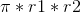, but for the Circle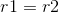, so the equation is the same as the standard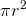. This loop will iterate through the objects, using the appropriate method in each case. Thus, you will get two values: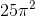, which is relatively close to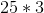or.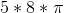, which is relatively close to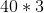or.

(Notice that the line numbers need to be "1" and "2".)

### Example Question #1 : Interface Declarations

Why is the "default" keyword used in a switch statement?

To catch any remaining possible values that may not be included in the case statements.

It defaults the case statement the case 1 if the function is stuck in an infinite loop.

It sets a variable to be a default value in case that value isn't defined in the case statement.

Default statements are unnecessary in case statements.

It makes the function default to a backup function if the case isn't present.

To catch any remaining possible values that may not be included in the case statements.

Explanation:

The default keyword is used to catch any remaining cases not specified. For example, if you only want to check for certain hour of the day, say 2,3, and 4 o'clock, the default case would catch all the other hours. You could then create a statement for what to do when those values are caught.

### Example Question #21 : Program Implementation

Which of the following declares an interface to be used for anything that can implement the actions of a sink?

public interface Sink {

public void Turn(int faucetNum);

public void Drain() {

// Drain code...

};

public void Autoclean(int setting);

}

public abstract class Sink {

abstract public void Turn(int faucetNum);

abstract public void Drain();

abstract public void Autoclean(int setting);

}

public abstract class Sink {

abstract public void Turn(int faucetNum);

public void Drain() {

// Drain code...

};

abstract public void Autoclean(int setting);

}

public interface Sink {

public void Turn(int faucetNum);

public void Drain();

public void Autoclean(int setting);

}

public interface Sink {

public void Turn(int faucetNum) {

// Turn code... excerpted

}

public void Drain() {

// Drain code... excerpted

};

abstract public void Autoclean(int setting) {

// Auto-clean code... excerpted

}

}

public interface Sink {

public void Turn(int faucetNum);

public void Drain();

public void Autoclean(int setting);

}

Explanation:

First of all, the question does ask for an interface.  This is a type of declaration in Java, so you should use that for your answer.  This allows you to eliminate two of the incorrect options.  Granting this, you then know that you need to look at the options that use the interface keyword.  Recall that for interfaces, you provide no implementation whatsoever.  Thus, the other two wrong answers are the ones that provide method bodies.  This cannot be done for interfaces, which merely define what something should do but do not actually implement it.

### Example Question #1 : Interface Declarations

Design an interface Cat. The interface must include the functions meow, purr, clean, hungry, isPurring, isClean, isSleeping, and isHungry.

interface Cat {

public String meow();

public String purr();

public void clean();

public void hungry();

public boolean isPurring();

public boolean isClean();

public boolean isSleeping();

public boolean isHungry();

}

class Cat {

public String meow();

public String purr();

public void clean();

public void hungry();

public boolean isPurring();

public boolean isClean();

public boolean isSleeping();

public boolean isHungry();

}

interface Cat {

public String meow();

public String purr();

public void clean();

public void hungry();

public void isPurring();

public void isClean();

public void isSleeping();

public void isHungry();

}

interface Cat {

}

interface Cat {

public String meow();

public String purr();

public void clean();

public void hungry();

public boolean isPurring();

public boolean isClean();

public boolean isSleeping();

public boolean isHungry();

}

Explanation:

The correct answer defines the interface as described. One of the answer choices defines a class with all of the attributes of the interface. Classes and interfaces are different. Interfaces are stubs of methods and classes are typically implementations (this is not always true, look up abstract classes). Another answer choice defines just the stub of the interface. This one would have been correct if the prompt had just asked for the stub, but there were implementation questions involved. Finally, the third answer choice had isPurring(), isClean(), isSleeping(), and isHungry() as void methods. These methods are prefixed with an "is" and "is" typically defines boolean language. Boolean was the correct return method on those.

### All AP Computer Science A Resources